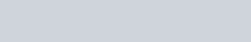If three metallic spheres of radii 6 cm,
Question:

If three metallic spheres of radii 6 cm, 8 cm and 10 cm are melted to form a single sphere, the diameter of the sphere is

(a) 12 cm

(b) 24 cm

(c) 30 cm

(d) 36 cm

Solution:

Let r be the radius of single sphere.

Now,

The volume of single sphere = sum of volume of three spheres

$\frac{4}{3} \pi r^{3}=\frac{4}{3} \pi(61)^{3}+\frac{4}{3} \pi(8)^{3}+\frac{4}{3} \pi(10)^{3}$$r^{3}=1728$

$r=12 \mathrm{~cm}$

Hence, the diameter = 20 × r = 24 cm

Hence, the correct answer is choice (b).